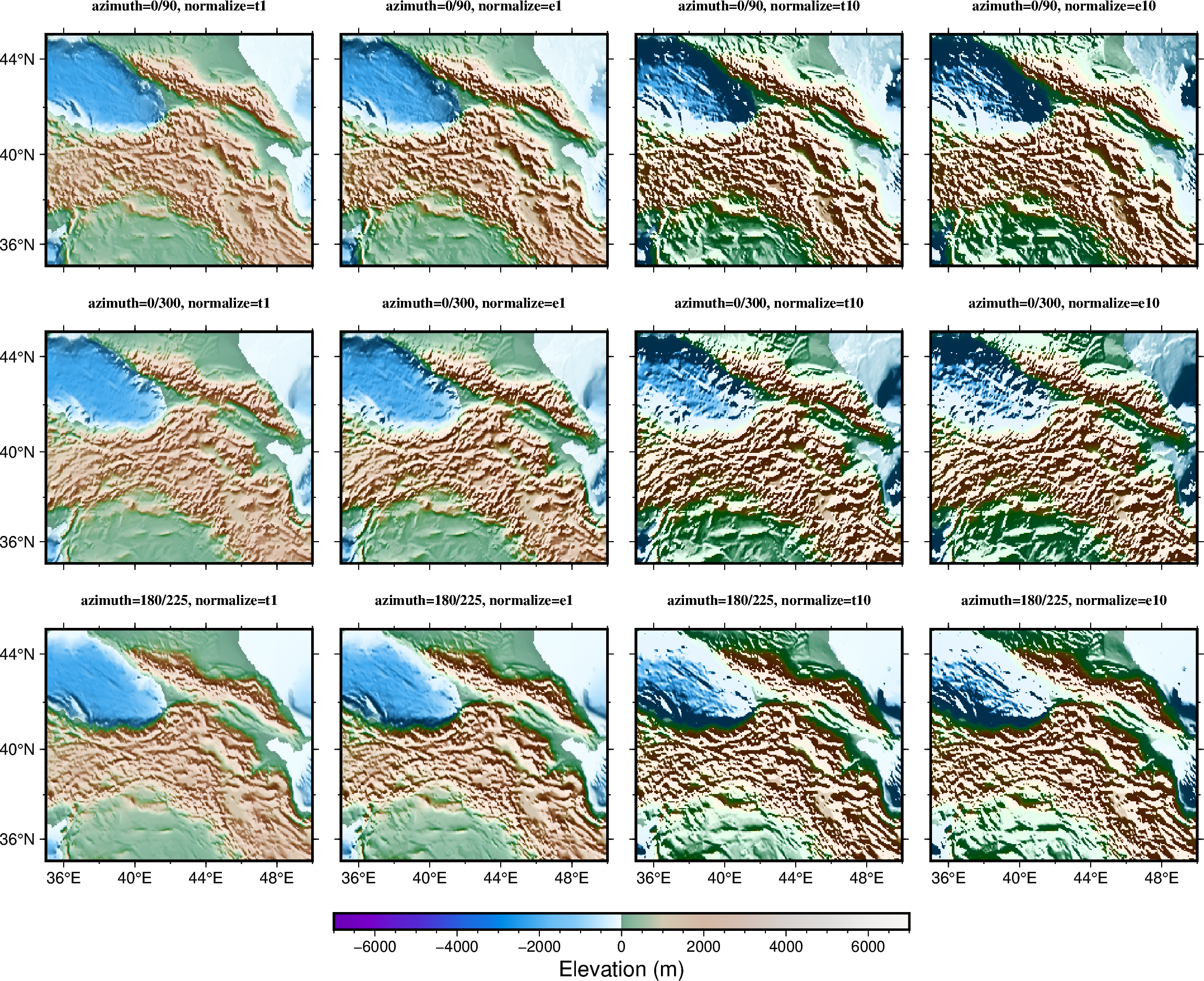# Calculating grid gradient with custom `azimuth` and `normalize` parameters

The `pygmt.grdgradient` function calculates the gradient of a grid file. As input, `pygmt.grdgradient` gets a `xarray.DataArray` object or a path string to a grid file. It then calculates the respective gradient and returns an `xarray.DataArray` object. The example below sets two main parameters:

• `azimuth`: to set the illumination light source direction (0° is North, 90° is East, 180° is South, 270° is West).

• `normalize` to enhance the three-dimensional sense of the topography.

The `normalize` parameter calculates the azimuthal gradient of each point along a certain azimuth angle, then adjusts the brightness value of the color according to the positive/negative of the azimuthal gradient and the amplitude of each point.```grdblend [NOTICE]: Remote data courtesy of GMT data server oceania [http://oceania.generic-mapping-tools.org]
grdblend [NOTICE]: SRTM15 Earth Relief v2.5.5 at 03x03 arc minutes reduced by Gaussian Cartesian filtering (5.6 km fullwidth) [Tozer et al., 2019].
```

```import pygmt

# Load the 3 arc-minutes global relief grid in the target area around Caucasus
grid = pygmt.datasets.load_earth_relief(resolution="03m", region=[35, 50, 35, 45])

fig = pygmt.Figure()

# Define a colormap to be used for topography
pygmt.makecpt(cmap="terra", series=[-7000, 7000])

# Define figure configuration
pygmt.config(FONT_TITLE="10p,5", MAP_TITLE_OFFSET="1p", MAP_FRAME_TYPE="plain")

# Setup subplot panels with three rows and four columns
with fig.subplot(
nrows=3,
ncols=4,
figsize=("28c", "21c"),
sharex="b",
sharey="l",
):
# E.g. "0/90" illuminates light source from the North (top) and East
# (right), and so on
for azi in ["0/90", "0/300", "180/225"]:
# "e" and "t" are cumulative Laplace distribution and cumulative
# Cauchy distribution, respectively
# "amp" (e.g. 1 or 10) controls the brightness value of the color
for nor in ["t1", "e1", "t10", "e10"]:
# Making an intensity DataArray using azimuth and normalize
# parameters
fig.grdimage(
grid=grid,
projection="M?",
frame=["a4f2", f"+tazimuth={azi}, normalize={nor}"],
cmap=True,
panel=True,
)

fig.colorbar(position="JBC+w10c/0.25c+h", frame="a2000f500+lElevation (m)")

fig.show()
```

Total running time of the script: ( 0 minutes 8.770 seconds)

Gallery generated by Sphinx-Gallery